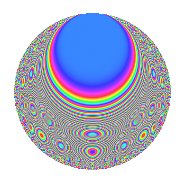# Properties

 Label 252.2.bbLevel 252 Weight 2 Character orbit bb Rep. character $$\chi_{252}(11,\cdot)$$ Character field $$\Q(\zeta_{6})$$ Dimension 88 Newform subspaces 1 Sturm bound 96 Trace bound 0

# Related objects

## Defining parameters

 Level: $$N$$ = $$252 = 2^{2} \cdot 3^{2} \cdot 7$$ Weight: $$k$$ = $$2$$ Character orbit: $$[\chi]$$ = 252.bb (of order $$6$$ and degree $$2$$) Character conductor: $$\operatorname{cond}(\chi)$$ = $$252$$ Character field: $$\Q(\zeta_{6})$$ Newform subspaces: $$1$$ Sturm bound: $$96$$ Trace bound: $$0$$

## Dimensions

The following table gives the dimensions of various subspaces of $$M_{2}(252, [\chi])$$.

Total New Old
Modular forms 104 104 0
Cusp forms 88 88 0
Eisenstein series 16 16 0

## Trace form

 $$88q - 2q^{4} - 6q^{5} - 6q^{6} - 2q^{9} + O(q^{10})$$ $$88q - 2q^{4} - 6q^{5} - 6q^{6} - 2q^{9} + 2q^{10} + 6q^{12} - 4q^{13} - 18q^{14} - 2q^{16} + 2q^{18} - 6q^{20} - 6q^{21} - 6q^{22} - 14q^{24} + 30q^{25} + 6q^{26} - 24q^{29} - 29q^{30} + 10q^{33} - 4q^{34} + 2q^{36} - 4q^{37} - 45q^{38} - 4q^{40} - 12q^{41} - 46q^{42} + 57q^{44} - 18q^{45} - 6q^{46} - 43q^{48} - 2q^{49} + 9q^{50} - 7q^{52} + 23q^{54} - 24q^{56} - 28q^{57} + 5q^{58} - 19q^{60} - 4q^{61} - 8q^{64} + 60q^{66} - 12q^{68} - 6q^{69} - 27q^{70} - 10q^{72} - 4q^{73} + 51q^{74} - 6q^{76} - 30q^{77} + 55q^{78} - 87q^{80} - 34q^{81} - 4q^{82} - 55q^{84} - 14q^{85} + 81q^{86} + 9q^{88} - 60q^{89} + 41q^{90} + 24q^{92} + 30q^{93} - 18q^{94} - 29q^{96} - 4q^{97} + 57q^{98} + O(q^{100})$$

## Decomposition of $$S_{2}^{\mathrm{new}}(252, [\chi])$$ into newform subspaces

Label Dim. $$A$$ Field CM Traces $q$-expansion
$$a_2$$ $$a_3$$ $$a_5$$ $$a_7$$
252.2.bb.a $$88$$ $$2.012$$ None $$0$$ $$0$$ $$-6$$ $$0$$

## Hecke Characteristic Polynomials

There are no characteristic polynomials of Hecke operators in the database